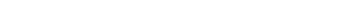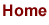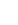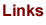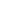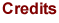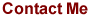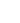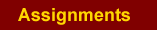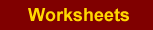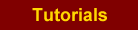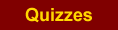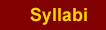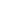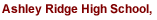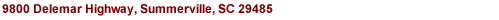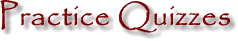Algebra 2 Pre-Calculus Chapters below are from PreCalculus: Graphical, Numerical, Algebraic (6th ed.) by Demana, Waits, Foley, Kennedy Chapter P Prerequisites Chapter 1: Functions & Graphs Chapter 2.1 - 2.4: Polynomial and Power Functions Chapter 2.5 - 2.9: Complex Zeros & Rational Functions Chapter 3: Exponential, Logistic, & Logarithmic Functions Chapter 9: Discrete Mathematics Chapter 4: Trigonometric Functions Chapter 5: Analytic Trigonometry Chapter 6: Vectors, Parametric Equations, and Polar Equations